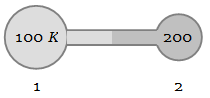NEET Physics Kinetic Theory of Gases Questions Solved

Figure shows two flasks connected to each other. The volume of the flask 1 is twice that of flask 2. The system is filled with an ideal gas at temperature 100 K and 200 K respectively. If the mass of the gas in 1 be m then what is the mass of the gas in flask 21. $\mathrm{m}$

2. $\frac{\mathrm{m}}{2}$

3. $\frac{\mathrm{m}}{4}$

4. $\frac{\mathrm{m}}{8}$

Explanation is a part of a Paid Course. To view Explanation Please buy the course.

Difficulty Level: